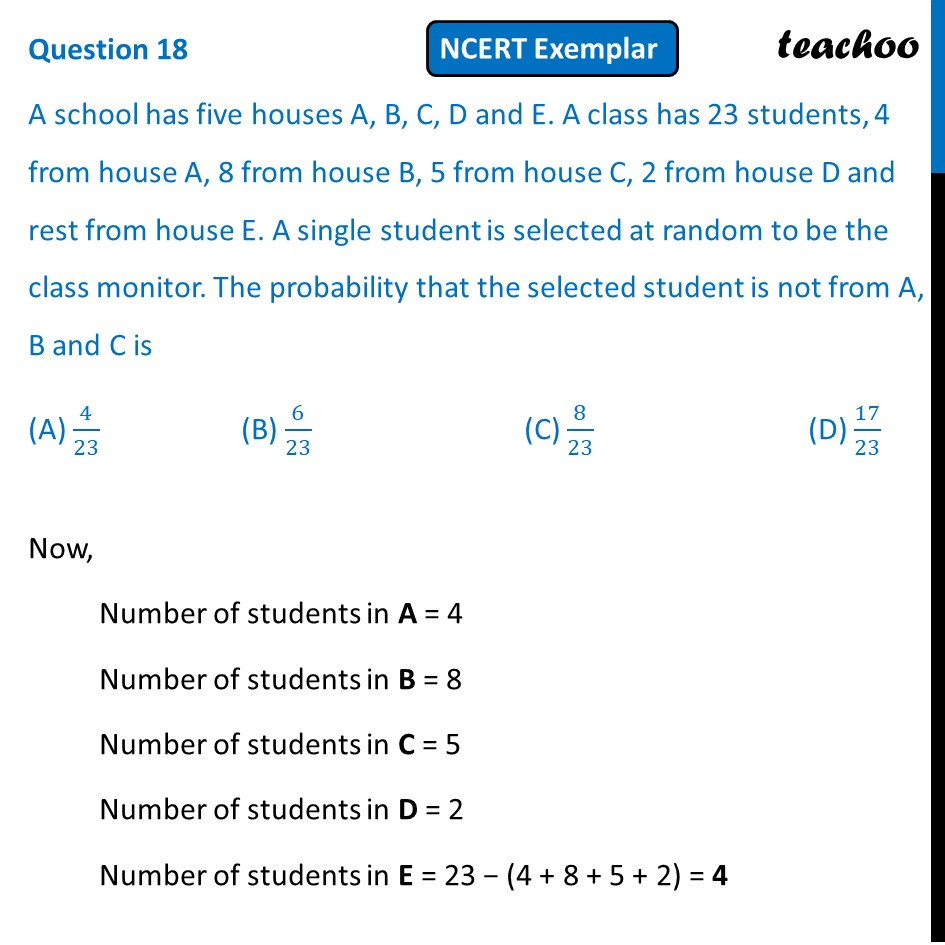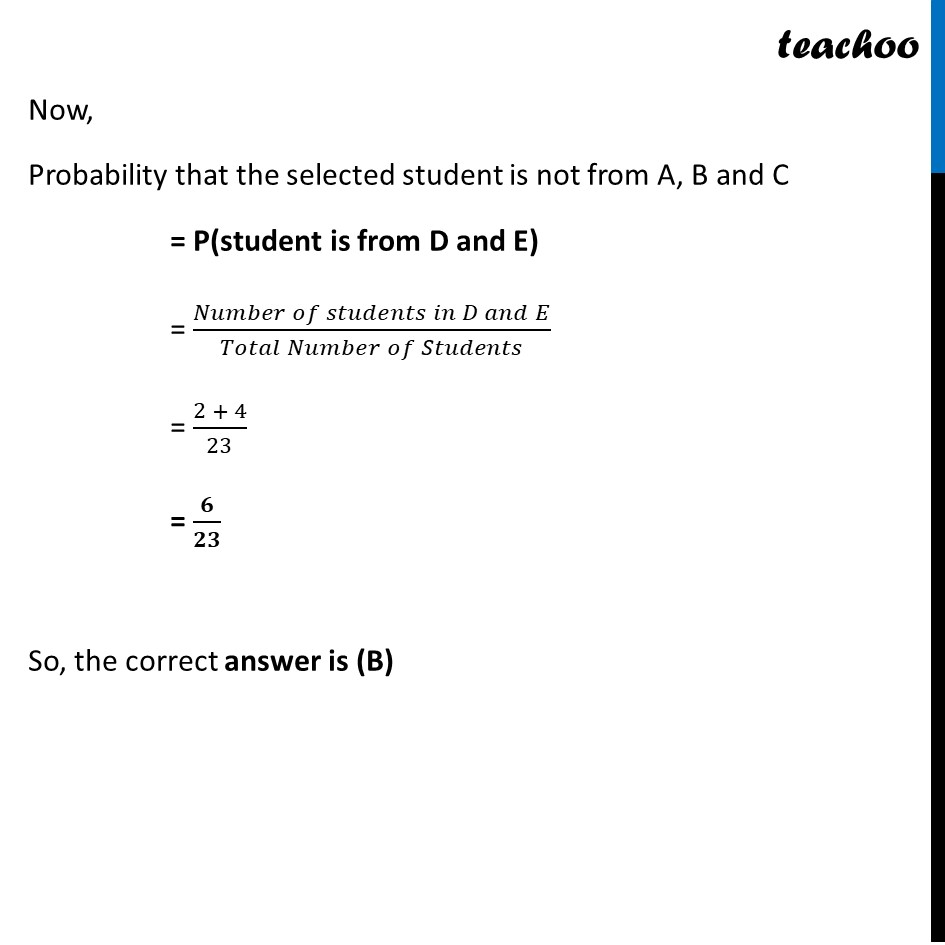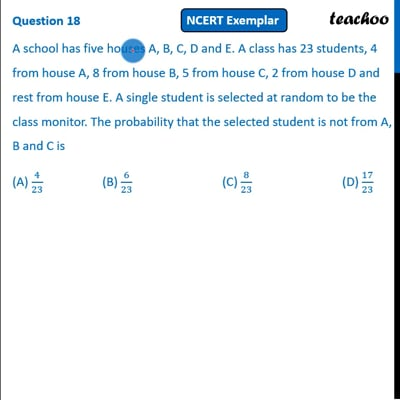NCERT Exemplar - MCQ

Chapter 15 Class 10 Probability
Serial order wise

## (D) 17/23This video is only available for Teachoo black users

Introducing your new favourite teacher - Teachoo Black, at only ₹83 per month

### Transcript

Question 18 A school has five houses A, B, C, D and E. A class has 23 students, 4 from house A, 8 from house B, 5 from house C, 2 from house D and rest from house E. A single student is selected at random to be the class monitor. The probability that the selected student is not from A, B and C is (A) 4/23 (B) 6/23 (C) 8/23 (D) 17/23 Now, Number of students in A = 4 Number of students in B = 8 Number of students in C = 5 Number of students in D = 2 Number of students in E = 23 − (4 + 8 + 5 + 2) = 4 Now, Probability that the selected student is not from A, B and C = P(student is from D and E) = (𝑁𝑢𝑚𝑏𝑒𝑟 𝑜𝑓 𝑠𝑡𝑢𝑑𝑒𝑛𝑡𝑠 𝑖𝑛 𝐷 𝑎𝑛𝑑 𝐸)/(𝑇𝑜𝑡𝑎𝑙 𝑁𝑢𝑚𝑏𝑒𝑟 𝑜𝑓 𝑆𝑡𝑢𝑑𝑒𝑛𝑡𝑠) = (2 + 4)/23 = 𝟔/𝟐𝟑 So, the correct answer is (B)